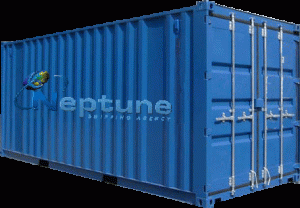•• # High Cube Container Height

The height of high cube containers iseither 40’ or 45’. However the capacities of these containers are dependent on the other specifications and features of the unit.

### 40’ High Cube Steel Containers

A 40’ high cube usually has an overall size of 40′ x 8′ x 9′ 6". The door opening width is 2,340 mm and the height is 2,577 mm. The internal dimensions are as follows.

The length is 12,035 mm, the width is 2,350 mm and the height to the load line is 2,697 mm. The maximum gross is 34,000 kg; the tare is 3,800 kg and the max payload is 30,200 kg. The capacity to the load line is 76 cubic meters.

### 45’ High Cube Steel Containers

When the height of high cube containers is 45’, the dimensions usually are 45′ x 8′ x 9′ 6". The door opening is 2,340 mm wide and 2,585 high. The internal dimensions are: 13,556 mm (length), 2,352 (width) and the height to load line is 2,697 mm.

The max gross is 32,500    kg and the tare is 4,800 kg. The maximum payload is 27,820 kg. The capacity to load line is 86 cubic meters.

### 40’ High Cube Aluminum Containers

A 40’ high container typically has dimensions of 40′ x 8′ x 9′ 6". The width of the door opening is 2,343 mm and the height is 2,584 mm. In terms of the interior dimensions, it is 12,056 mm long, 2,347 mm wide and the height to the load line is 2,684 mm.

The height of high cube containers like this gives it a maximum gross of 32,500 kg. The tare is 2,900 kg and the maximum payload is 29,600 kg. The capacity to load line is usually 76 cubic meters.

### 45’ High Cube Aluminum Containers

The typical dimensions of these containers are 45′ x 8′ x 9′ 6". The width of the door opening is 2,340 mm and the height is 2,584 mm. Internally, the container is 13,582 mm long, 2,347 wide and the height to load line is 2,696 mm.

The maximum gross is 32,500 kg and the tare is 3,900 kg. The maximum payload is 28,600 kg. The capacity to load line is 86 cubic meters.

### 40’ High Cube Reefer Containers

The height of high cube containers in the reefer variety is usually 40’. The dimensions are 40′ x 8′ x 9′ 6". The door opening is 2,278 mm wide and 2,473 mm high.

The internal dimensions are 11,578 mm (length), 2,280 (width) and the height to load line is 2,425 mm. The maximum gross is 34,000 kg and the tare is 4,500 kg. The maximum payload is 29,500 kg and the capacity to load line is 64 cubic meters.

When the reefer is 45’ high, the rest of the dimensions are typically 45′ x 8′ x 9′ 6". The width of the door opening is 2,340 mm and the height is 2,584 mm. The height of high cube containers will also affect the specs of other types of storage devices.